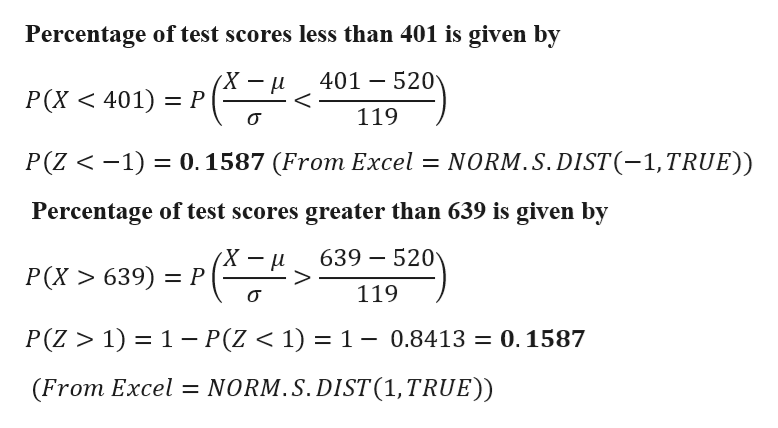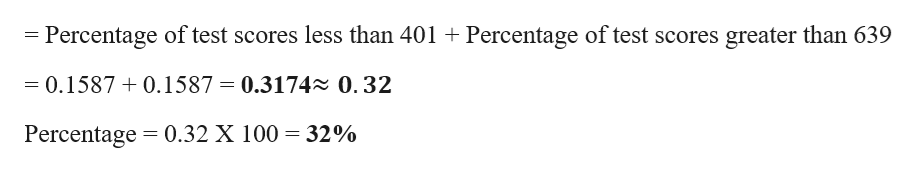# A certain standardized​ test's math scores have a​ bell-shaped distribution with a mean of 520 and a standard deviation of 119.What percentage of standardized test scores is less than 401 or greater than 639​?

Question
7 views

A certain standardized​ test's math scores have a​ bell-shaped distribution with a mean of 520 and a standard deviation of 119.

What percentage of standardized test scores is less than 401 or greater than 639​?

check_circle

Step 1

Given Data

Mean = 520

Standard Deviation = 119

Percentage of test scores less than 401 is given byhelp_outlineImage TranscriptionclosePercentage of test scores less than 401 is given by 401 520 _ P(X 401) P 119 0.1587 (From Excel NORM.S. DIST (-1, TRUE)) P(Z <1) Percentage of test scores greater than 639 is given by 639 520 P(X639) P 119 P(Z > 1) 1- P(Z < 1) 1 - 0.8413 = 0.1587 NORM.S. DIST (1, TRUE)) (From Excel fullscreen
Step 2

Percentage of standardized test scores is less than ...help_outlineImage Transcriptionclose= Percentage of test scores less than 401 Percentage of test scores greater than 639 = 0.1587 0.1587 = 0.3174 0.32 Percentage 0.32 X 100 32% fullscreen

### Want to see the full answer?

See Solution

#### Want to see this answer and more?

Solutions are written by subject experts who are available 24/7. Questions are typically answered within 1 hour.*

See Solution
*Response times may vary by subject and question.
Tagged in

### Statistics